Courses

# Questions with Answers: Analogy SSC Notes | EduRev

## SSC: Questions with Answers: Analogy SSC Notes | EduRev

The document Questions with Answers: Analogy SSC Notes | EduRev is a part of the SSC Course General Aptitude for GATE.
All you need of SSC at this link: SSC

Directions to Solve
Each of the following questions consists of two sets of figures. Figures A, B, C and D constitute the Problem Set while figures 1, 2, 3, 4 and 5 constitute the Answer Set. There is a definite relationship between figures A and B. Establish a similar relationship between figures C and D by selecting a suitable figure from the Answer Set that would replace the question mark (?) in fig. (D).

Question 1)
Select a suitable figure from the Answer Figures that would replace the question mark (?).
Problem Figures: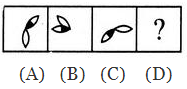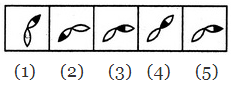A. 1
B. 2
C. 3
D. 4
E. 5
Solution:
The half-shaded leaf rotates 135ºAnti Clock Wise and the un-shaded leaf rotates 135ºClock Wise.

Question 2)
Select a suitable figure from the Answer Figures that would replace the question mark (?).
Problem Figures: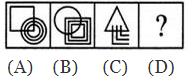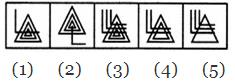A. 1
B. 2
C. 3
D. 4
E. 5
Solution:
The upper element is converted to an element similar to the lower elements and each one of the lower elements is converted to an element similar to the upper element.

Question 3)
Select a suitable figure from the Answer Figures that would replace the question mark (?).
Problem Figures: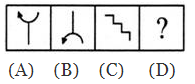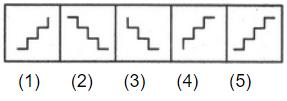A. 1
B. 2
C. 3
D. 4
E. 5
Solution: The figure gets vertically inverted.

Question 4) Select a suitable figure from the Answer Figures that would replace the question mark (?).
Problem Figures: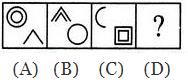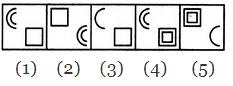Solution: Each one of the upperelementsisreplaced by an element similar to the lower element(s) and each one of the lower elements is replaced by an element similar to the upper element(s).

Question 5)
Select a suitable figure from the Answer Figures that would replace the question mark (?).
Problem Figures: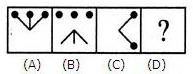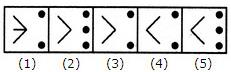Solution:
Except for the dots, the remaining part of the figure rotates through 180o and shifts to the opposite side of the square boundary.

The document Questions with Answers: Analogy SSC Notes | EduRev is a part of the SSC Course General Aptitude for GATE.
All you need of SSC at this link: SSCUse Code STAYHOME200 and get INR 200 additional OFF Use Coupon Code
All Tests, Videos & Notes of SSC: SSC

### Up next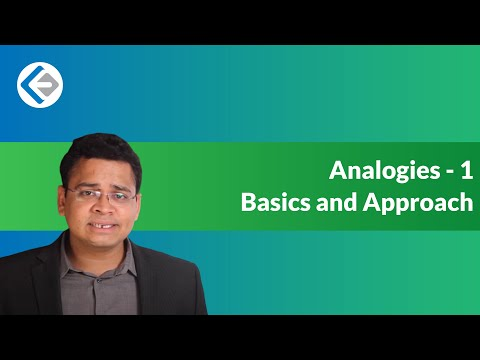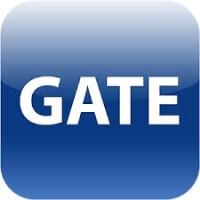## General Aptitude for GATE

61 videos|67 docs|88 tests

### Up nextTrack your progress, build streaks, highlight & save important lessons and more!

,

,

,

,

,

,

,

,

,

,

,

,

,

,

,

,

,

,

,

,

,

;